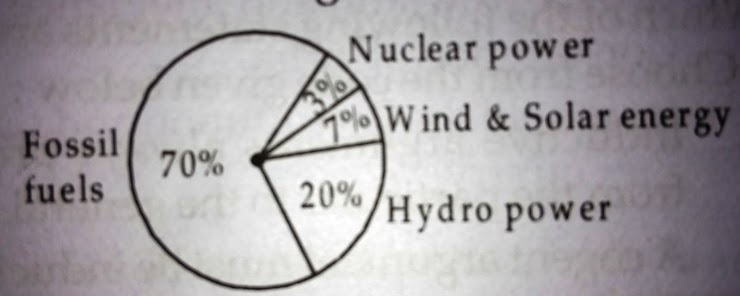UGC-NET/SET-PAPER-I(2012-JUNE) - MODEL PAPER-122
ONLINE TEST NET/SET PAPER-1
Email address *
21. If Ram knows that y is an integer greater than 2 and less than 7 and Hari knows that y is an integer greater than 5 and less than 10, then they may correctly conclude that
2 points
22. Four pipes can fill a reservoir in 15, 20, 30 and 60 hours respectively. The first one was opened at 6 AM, second at 7 AM, third at 8 AM and the fourth at 9 AM. When will the reservoir be filled ?
2 points
The total electricity generation in a country is 97 GW. The contribution of various energy sources is indicated in percentage terms in the Pie Chart given below:
2 points24. What is the contribution of renewable energy sources in absolute terms in the electricity generation?
2 points
25. TCP/IP is necessary if one is to connect to the
2 points
26. Each character on the keyboard of computer has an ASCII value which stands for
2 points
27. Which of the following is not a programming language?
2 points
28. Minimum number of bits required to store any 3 digit decimal number is equal to
2 points
29. Internet explorer is a type of
2 points
30. POP3 and IMAP are e-mail accounts in which
2 points